# Topics

AlignmentSuperimposing the symbols of one string over those of another (with gap symbols inserted into the strings) to represent insertions, deletions, and substitutions between the strings.

0 / 19

Problems Solved

Combinatorics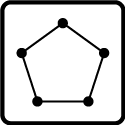The mathematics of counting objects.

0 / 22

Problems Solved

Computational Mass SpectrometryA technique used to identify molecules by splitting them into pieces and analyzing chemical properties of these small pieces.

0 / 6

Problems Solved

Divide-and-conquer0 / 2

Problems Solved

Dynamic ProgrammingThe algorithmic notion of building up a solution to a problem by solving it on progressively larger cases.

0 / 23

Problems Solved

Genome Assembly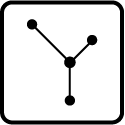The algorithmic reconstruction of contiguous chromosomes from short fragments of DNA.

0 / 7

Problems Solved

Genome Rearrangements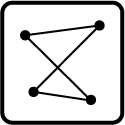A large-scale mutation that affects the makeup of entire intervals of nucleic acid.

0 / 6

Problems Solved

Graph Algorithms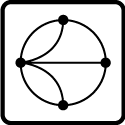An algorithm requiring the interpretation and manipulation of networks, or graphs.

0 / 11

Problems Solved

GraphsA network containing a collection of nodes, pairs of which are joined by edges.

0 / 18

Problems Solved

Heredity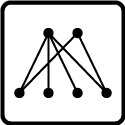The scientific study of the inheritance of traits.

0 / 6

Problems Solved

Phylogeny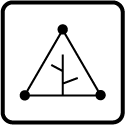A tree modeling the evolutionary scenario deriving a collection of taxa from their proposed ancestors.

0 / 19

Problems Solved

Population Dynamics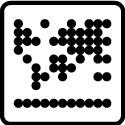0 / 3

Problems Solved

Probability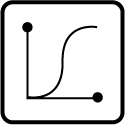The mathematical study of the chance of occurrence of random events, or the chance with which a specific event will occur.

0 / 13

Problems Solved

Set Theory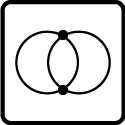The mathematical study of sets and their properties.

0 / 3

Problems Solved

SortingThe problem of finding a smallest collection of operations that changes a structure into a nicely ordered one.

0 / 14

Problems Solved

String AlgorithmsAn algorithm involving the manipulation and properties of chains of symbols.

0 / 26

Problems Solved